Courses

# Shortest Paths MCQ - 1

## 20 Questions MCQ Test RRB JE for Computer Science Engineering | Shortest Paths MCQ - 1

Description
This mock test of Shortest Paths MCQ - 1 for Computer Science Engineering (CSE) helps you for every Computer Science Engineering (CSE) entrance exam. This contains 20 Multiple Choice Questions for Computer Science Engineering (CSE) Shortest Paths MCQ - 1 (mcq) to study with solutions a complete question bank. The solved questions answers in this Shortest Paths MCQ - 1 quiz give you a good mix of easy questions and tough questions. Computer Science Engineering (CSE) students definitely take this Shortest Paths MCQ - 1 exercise for a better result in the exam. You can find other Shortest Paths MCQ - 1 extra questions, long questions & short questions for Computer Science Engineering (CSE) on EduRev as well by searching above.
QUESTION: 1

### Consider the directed graph shown in the figure below. There are multiple shortest paths between vertices S and T. Which one will be reported by Dijstra?s shortest path algorithm? Assume that, in any iteration, the shortest path to a vertex v is updated only when a strictly shorter path to v is discovered.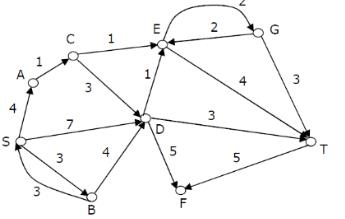Solution:

See Dijkstra’s shortest path algorithm When the algorithm reaches vertex 'C', the distance values of 'D' and 'E' would be 7 and 6 respectively. So the next picked vertex would be 'E'

QUESTION: 2

### To implement Dijkstra’s shortest path algorithm on unweighted graphs so that it runs in linear time, the data structure to be used is:

Solution:

The shortest path in an un-weighted graph means the smallest number of edges that must be traversed in order to reach the destination in the graph. This is the same problem as solving the weighted version where all the weights happen to be 1. If we use Queue (FIFO) instead of Priority Queue (Min Heap), we get the shortest path in linear time O(|V| + |E|). Basically we do BFS traversal of the graph to get the shortest paths.

QUESTION: 3

### Dijkstra’s single source shortest path algorithm when run from vertex a in the below graph, computes the correct shortest path distance to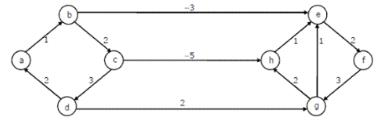Solution:

Dijkstra’s single source shortest path is not guaranteed to work for graphs with negative weight edges, but it works for the given graph. Let us see... Let us run the 1st pass b 1 b is minimum, so shortest distance to b is 1. After 1st pass, distances are c 3, e -2. e is minimum, so shortest distance to e is -2 After 2nd pass, distances are c 3, f 0. f is minimum, so shortest distance to f is 0 After 3rd pass, distances are c 3, g 3. Both are same, let us take g. so shortest distance to g is 3. After 4th pass, distances are c 3, h 5 c is minimum, so shortest distance to c is 3 After 5th pass, distances are h -2 h is minimum, so shortest distance to h is -2

QUESTION: 4

In an unweighted, undirected connected graph, the shortest path from a node S to every other node is computed most efficiently, in terms of time complexity by

Solution:

* Time Comlexity of the Dijkstra’s algorithm is O(|V|^2 + E)
* Time Comlexity of the Warshall’s algorithm is O(|V|^3) *
DFS cannot be used for finding shortest paths
* BFS can be used for unweighted graphs. Time Complexity for BFS is O(|E| + |V|)

QUESTION: 5

Suppose we run Dijkstra’s single source shortest-path algorithm on the following edge weighted directed graph with vertex P as the source. In what order do the nodes get included into the set of vertices for which the shortest path distances are finalized?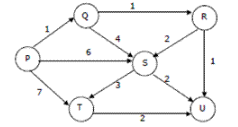Solution:
QUESTION: 6

What is the time complexity of Bellman-Ford single-source shortest path algorithm on a complete graph of n vertices?

Solution:

Time complexity of Bellman-Ford algorithm is θ(VE) where V is number of vertices and E is number edges (See this). If the graph is complete, the value of E becomes θ(V^2). So overall time complexity becomes θ(V^3)

QUESTION: 7

In a weighted graph, assume that the shortest path from a source 's' to a destination 't' is correctly calculated using a shortest path algorithm. Is the following statement true? If we increase weight of every edge by 1, the shortest path always remains same.

Solution:

See the following counterexample. There are 4 edges s-a, a-b, b-t and s-t of wights 1, 1, 1 and 4 respectively. The shortest path from s to t is s-a, a-b, b-t. IF we increase weight of every edge by 1, the shortest path changes to s-t.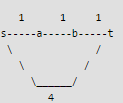QUESTION: 8

Following statement is true or false?
If we make following changes to Dijkstra, then it can be used to find the longest simple path, assume that the graph is acyclic.

1) Initialize all distances as minus infinite instead of plus infinite.
2) Modify the relax condition in Dijkstra's algorithm to update distance of an adjacent v of the currently considered vertex u only if "dist[u]+graph[u][v] > dist[v]". In shortest path algo, the sign is opposite.

Solution:

In shortest path algo, we pick the minimum distance vertex from the set of vertices for which distance is not finalized yet. And we finalize the distance of the minimum distance vertex. For maximum distance problem, we cannot finalize the distance because there can be a longer path through not yet finalized vertices.

QUESTION: 9

Which of the following algorithm can be used to efficiently calculate single source shortest paths in a Directed Acyclic Graph?

Solution:

Using Topological Sort, we can find single source shortest paths in O(V+E) time which is the most efficient algorithm. See following for details. Shortest Path in Directed Acyclic Graph

QUESTION: 10

Given a directed graph where weight of every edge is same, we can efficiently find shortest path from a given source to destination using?

Solution:

With BFS, we first find explore vertices at one edge distance, then all vertices at 2 edge distance, and so on.

QUESTION: 11

Is the following statement valid about shortest paths? Given a graph, suppose we have calculated shortest path from a source to all other vertices. If we modify the graph such that weights of all edges is becomes double of the original weight, then the shortest path remains same only the total weight of path changes.

Solution:

The shortest path remains same. It is like if we change unit of distance from meter to kilo meter, the shortest paths don't change.

QUESTION: 12

Match the following

Group A                                                                        Group B
a) Dijkstra's single shortest path algo                      p) Dynamic Programming
b) Bellmen Ford's single shortest path algo             q) Backtracking
c) Floyd Warshell's all pair shortest path algo.         r) Greedy Algorithm

Solution:

Dijkstra is a greedy algorithm where we pick the minimum distant vertex from not yet finalized vertices. Bellman Ford and Floyd Warshell both are Dynamic Programming algorithms where we build the shortest paths in bottom up manner.

QUESTION: 13

Is the following statement valid?.

Given a weighted graph where weights of all edges are unique (no two edge have same weights), there is always a unique shortest path from a source to destination in such a graph.

Solution:

There can be more than one paths with same weight. Consider a path with one edge of weight 5 and another path with two edges of weights 2 and 3. Both paths have same weights.

QUESTION: 14

Is the following statement valid?.
Given a graph where all edges have positive weights, the shortest paths produced by Dijsktra and Bellman Ford algorithm may be different but path weight would always be same.

Solution:

Dijkstra and Bellman-Ford both work fine for a graph with all positive weights, but they are different algorithms and may pick different edges for shortest paths.

QUESTION: 15

Which of the following statement(s)is / are correct regarding Bellman-Ford shortest path algorithm?

P: Always finds a negative weighted cycle, if one exist s.
Q: Finds whether any negative weighted cycle is reachable from the source.

Solution:

Bellman-Ford shortest path algorithm is a single source shortest path algorithm. So it can only find cycles which are reachable from a given source, not any negative weight cycle. Consider a disconnected where a negative weight cycle is not reachable from the source at all. If there is a negative weight cycle, then it will appear in shortest path as the negative weight cycle will always form a shorter path when iterated through the cycle again and again.

QUESTION: 16

Let G(V, E) an undirected graph with positive edge weights. Dijkstra's single-source shortest path algorithm can be implemented using the binary heap data structure with time complexity:

Solution:
QUESTION: 17

Let G = (V, E) be an undirected graph with a subgraph G1 = (V1, El). Weights are assigned to edges of G as follows :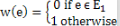A single-source shortest path algorithm is executed on the weighted graph (V, E, w) with an arbitrary vertex ν1 of V1 as the source. Which of the following can always be inferred from the path costs computed?

Solution:

When shortest path shortest path from v1 (one of the vertices in V1) is computed. G1 is connected if the distance from v1 to any other vertex in V1 is greater than 0, otherwise G1 is disconnected.

QUESTION: 18

Let G (V, E) be a directed graph with n vertices. A path from vi to vj in G is sequence of vertices (vi, vi+1, ......., vj) such that (vk, vk+1) ∈ E for all k in i through j - 1. A simple path is a path in which no vertex appears more than once. Let A be an n x n array initialized as follow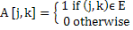Consider the following algorithm.

for i = 1 to n
for j = 1 to n
for k = 1 to n
A [j , k] = max (A[j, k] (A[j, i] + A [i, k]);

Q. Which of the following statements is necessarily true for all j and k after terminal of the above algorithm ?

Solution:

In the original input matrix, A[j , k] is 1 if there is an edge from j to k, else 0.
Below expression is important to note:
A[j, k] = max(A[j, k] (A[j, i] + A [i, k]);
This expression puts the count of maximum edges on a path from j to k. In this expression, we consider every vertex k that can become an intermediate vertex and can give longer path.

QUESTION: 19

Let G = (V, E) be a simple undirected graph, and s be a particular vertex in it called the source. For x ∈ V, let d(x) denote the shortest distance in G from s to x. A breadth first search (BFS) is performed starting at s. Let T be the resultant BFS tree. If (u, v) is an edge of G that is not in T, then which one of the following CANNOT be the value of d(u) – d(v)?

Solution:

Note that the given graph is undirected, so an edge (u, v) also means (v, u) is also an edge. Since a shorter path can always be obtained by using edge (u, v) or (v, u), the difference between d(u) and d(v) can not be more than 1.

QUESTION: 20

Let G be a directed graph whose vertex set is the set of numbers from 1 to 100. There is an edge from a vertex i to a vertex j iff either j = i + 1 or j = 3i. The minimum number of edges in a path in G from vertex 1 to vertex 100 is

Solution:

The task is to find minimum number of edges in a path in G from vertex 1 to vertex 100 such that we can move to either i+1 or 3i from a vertex i.
Since the task is to minimize number of edges, we would prefer to follow 3*i.
Let us follow multiple of 3.

1 => 3 => 9 => 27 => 81, now we can't follow multiple of 3. So we will have to follow i+1. This solution gives a long path.

What if we begin from end, and we reduce by 1 if the value is not multiple of 3, else we divide by 3.
100 => 99 => 33 => 11 => 10 => 9 => 3 => 1

So we need total 7 edges.